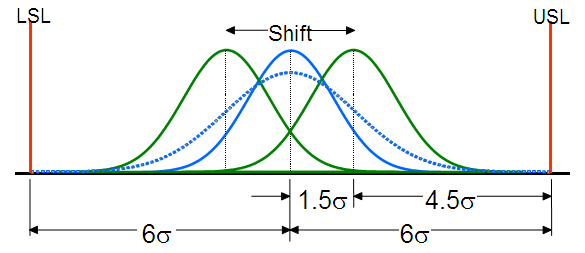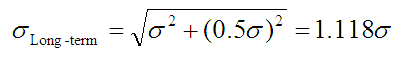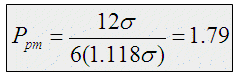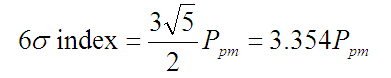# Six Sigma vs. ppm

The following shows how the Six Sigma Index compares with Process Performance and the ppm Index.Assume the true mean randomly shifts with a standard deviation of 0.5σ thenAssuming the process is centered within the specifications (ppm will equal pp). ppm can be calculated using the long-term sigma, 1.118σ, as follows. Our spec range, per the above diagram is 12σ.A ppm of 1.79 could be considered equivalent to Six Sigma.

The following table shows an equivalent Ppm for various values of the Six Sigma Index.

ppm6σ Index
0.893
1.194
1.495
1.796
2.097
2.398
2.689
2.9810